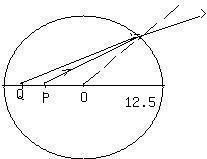A fish is swimming in water inside a thin spherical glass bowlof uniform thickness. Assuming the radius of curvature of thebowl is 25cm, locate the imChesley 2021-04-13 Answered
A fish is swimming in water inside a thin spherical glass bowlof uniform thickness. Assuming the radius of curvature of thebowl is 25cm, locate the image of the fish if the fish is located:(a) at the center of the bowl;
(b) 20.0 cm from the side of the bowl between the observer andthe center of the bowl.

• Questions are typically answered in as fast as 30 minutes

Solve your problem for the price of one coffee

• Math expert for every subject
• Pay only if we can solve itFaiza Fuller$$Ln_w=1.33, \ n_a=1$$
are the refreactive indices of water and air respectively.
When fish is at the center of bowl, $$\displaystyle{\frac{{{n}_{{w}}}}{{{p}}}}+{\frac{{{n}_{{a}}}}{{{q}}}}={\frac{{{n}_{{a}}-{n}_{{w}}}}{{{R}}}}$$
p is object q is image of fish and R is radius of curvature
$$\displaystyle{\frac{{{1.33}}}{{{12.5}}}}+{\frac{{{1}}}{{{q}}}}={\frac{{{1}-{1.33}}}{{-{12.5}}}}$$
$$\displaystyle{\frac{{{1}}}{{{q}}}}={\frac{{{.33}}}{{{12.5}}}}-{\frac{{{1.33}}}{{{12.5}}}}=-{\frac{{{1}}}{{{12.5}}}}$$
q=12.5 ie at the center itself
when fish is at 20 cm
$$\displaystyle{\frac{{{1.33}}}{{{20}}}}+{\frac{{{1}}}{{{q}}}}={\frac{{{1}-{1.33}}}{{-{12.5}}}}$$
$$\displaystyle{\frac{{{1}}}{{{q}}}}={\frac{{{.33}}}{{{12.5}}}}-{\frac{{{1.33}}}{{{20}}}}$$
q=24.9376cm
In this problem because glass is thin we neglect glassref index and take only water ref index and tha of air